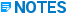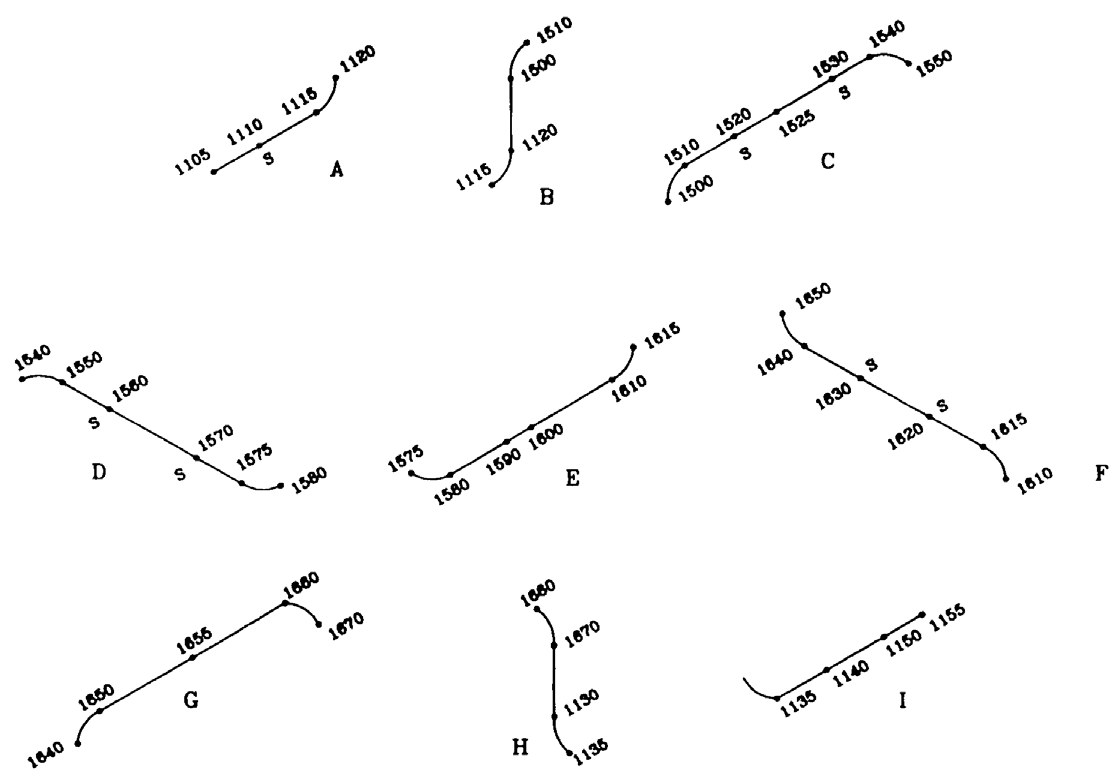# Node Layout - CAESAR II - Reference Data

## CAESAR II Applications Guide

PPMProduct
CAESAR II
PPMCategory_custom
Reference Data
Version_CAESAR
12

The system as defined in the preceding figure consists of nine segments of piping. Each segment is shown in the following figure with the node numbers assigned to the various points for the core piping. Each segment is discussed individually.• The term segment is used solely to assist in discussing this example.

• CAESAR II does not require the segregation of a piping system into segments. There are no such input requirements or restrictions in CAESAR II.• Segment A - This segment runs from the pump to the first elbow. Because this section is at ground level, the 100 series nodes are used. Because the pump acts as an anchor, the start node of this segment ends in 5, so the pump is assigned node 1105. The length of the segment requires an intermediate node point for a spider, so node 1110 is assigned 5-feet from the pump. Nodes 1120 and 1115 are assigned to the elbow. The positive Y support is not at node 1115 because 1115 is part of the core piping. The positive Y is applied at node 2115 (the jacket), so we assign the 5 to this node point.

• Segment B - This segment is the six-foot vertical section, beginning with the elbow at node 1120. This section can be modeled by coding to the top elbow and assigning nodes 1500 and 1510. The 500 series nodes are used here because the 2nd level piping is now being modeled.

• Segment C - The first horizontal run in the 2nd level requires a node at mid-span to accommodate a spring hanger (on the jacket). This mid-span node divides the segment into two 9-foot lengths, which exceed the maximum spider spacing of 6-feet. Therefore, the eighteen-foot span is divided into four elements, each one 4-feet, 6-inches. The nodes assigned are 1520, 1525 (for the hanger location), and 1530. The segment is finished off with the elbow modeled by nodes 1540 and 1550.

• Segment D - This horizontal segment in the 2nd level is modeled using nodes 1560, 1570, and nodes 1575 and 1580 at the elbow. The nodes 1560 and 1570 are for spiders, while 1575 is a hanger location

• Segment E - This horizontal segment contains the valve. Nodes for this segment are 1590, 1600, 1610, and 1615. Node 1615 terminates the elbow and is also a hanger location. The element from 1590 to 1600 should be declared rigid with a weight of 452 pounds. Starting with the elbow at nodes 1610 through 1615, all of the elbows are modeled as individual elements. This eases the subsequent coding of the jacket. The elbows in this part of the model consist of two straight pieces of pipe, equal in length to the radius of the elbow.

• Segment F -The third horizontal leg of the expansion loop is modeled using nodes 1620, 1630, 1640, and 1650.

• Segment G -The last horizontal run of the 2nd level is modeled using nodes 1655, 1660, and 1670. Node 1655 is a hanger location.

• Segment H - The second vertical section of piping returns the system to ground level. The only additional nodes required for this section are for the elbow at nodes 1130 and 1135. Node 1135 is a positive Y location on the jacket.

• Segment I - This is the last segment that terminates at the vessel nozzle. The nodes used to model this segment are 1140, 1150, and 1155.